# Precalculus - Circles

## Brief Introduction to Conic Sections and Circles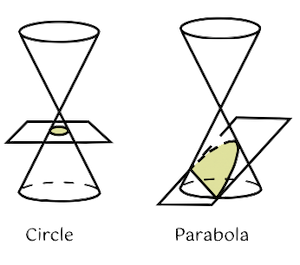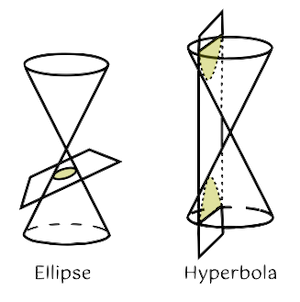• The figures are a group of curves called Conic Sections. They are formed from the intersection of a plane and right circular cones. Notice in the figures that they do not intersect the vertex of the cone.
• Basically, there are four types of conic sections - Circle, Parabola, Ellipse, and Hyperbola.
• In general, conic sections are represented by second-degree equations. This is written as $A{x}^{2}+Bxy+C{y}^{2}+Dx+Ey+F=0$ where A and C are nonzero integers.
• Considering the general form $A{x}^{2}+Bxy+C{y}^{2}+Dx+Ey+F=0$, if , then the graph of this equation represents a CIRCLE.
• A circle is a locus moving in a plane in such a way that its distance from a fixed point called the center remains constant.
• A circle is a special kind of ellipse.
• A circle is formed when a cone is cut perpendicular to its axis.

The equation of the circle where the center is  $\left(0,0\right)$ is given by ${x}^{2}+{y}^{2}={r}^{2}$ where $r=radius$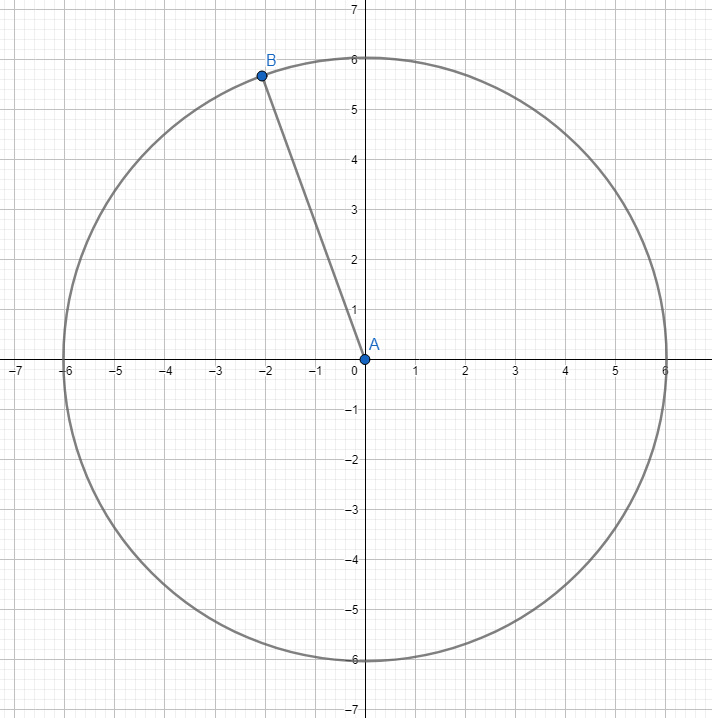The equation of the circle whose center is at $C\left(h,k\right)$ is given by ${\left(x-h\right)}^{2}+{\left(y-k\right)}^{2}={r}^{2}$ where $r=radius$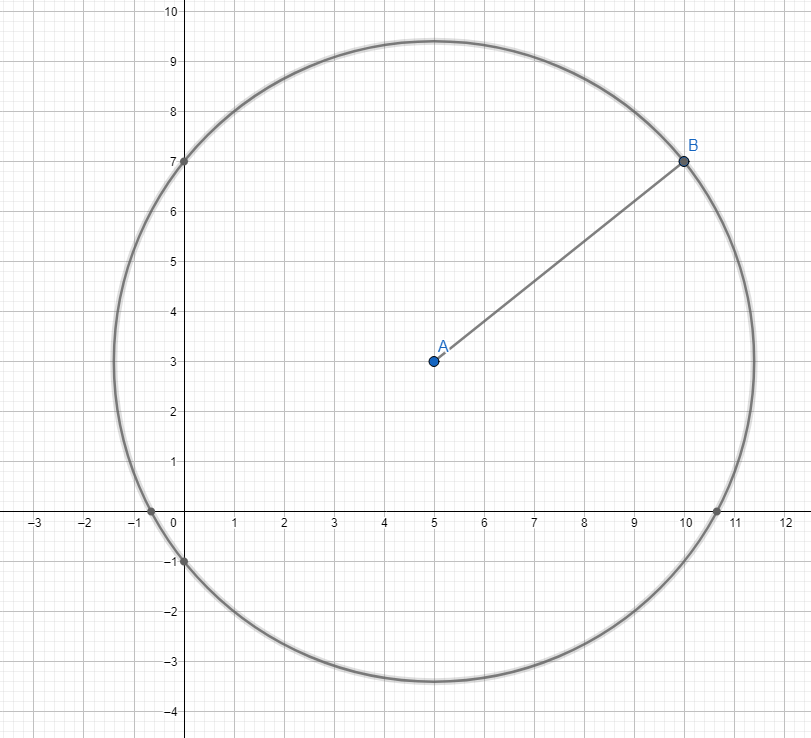The equation of the circle whose radius is equal to 1 and center at $\left(0,0\right)$ is given by ${x}^{2}+{y}^{2}=1$. This is called the Unit Circle.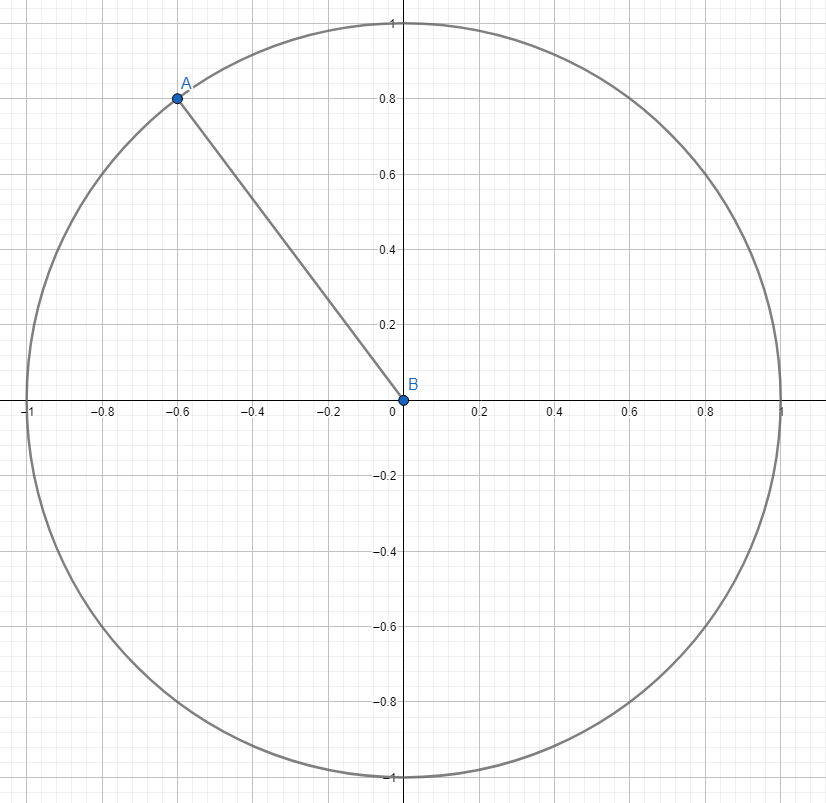## Solved Examples

Example 1. Find the center and radius of the circle represented by ${x}^{2}-8x-6y+{y}^{2}-11=0$.

Solution:

Using completing the square, we can solve for the coordinates of the center $C\left(h,k\right)$ and the radius.

${x}^{2}-8x+{y}^{2}-6y-11=0$

${x}^{2}-8x+16+{y}^{2}-6y+9=11+16+9$

${\left(x-4\right)}^{2}+{\left(y-3\right)}^{2}=36$

Hence the center is at $C\left(4,3\right)$ with a radius of $r=\sqrt{10}$

Example 2. What is the equation of the circle whose center is at $C\left(5,7\right)$ and passes through $\left(2,8\right)$?

Solution:

In this case, we need to solve for the radius using the distance formula:

${r}^{2}={\left(5-2\right)}^{2}+{\left(7-8\right)}^{2}$

${r}^{2}=9+1\to r=\sqrt{10}$

Thus, the equation of the circle is ${\left(x-5\right)}^{2}+{\left(y-7\right)}^{2}=10$.

Example 3. What is the equation of the circle that passes through the points ?

Solution:

Use the general equation of the circle $A{x}^{2}+C{y}^{2}+Dx+Ey+F=0$ where A = C = 1.

This forms a linear system $\left\{\begin{array}{l}-3D-9E+F=-90\\ 2D-4E+F=-20\\ 6D-6E+F=-72\end{array}\right\$

Solving the system yields

Substitute the obtained values to the general equation ${x}^{2}+{y}^{2}+Dx+Ey+F=0$.

Thus, the general equation of the circle is ${x}^{2}-4x+{y}^{2}+18y+60=0$.

The standard form equation is ${\left(x-2\right)}^{2}+{\left(y+9\right)}^{2}=25$.

## More Problems involving Circles, Tangents, and Radical Axis

Example 1. What is the equation of the circle whose center is at $C\left(-3,-4\right)$ a tangent to the line $3x+4y=15$?

Solution:

We need to apply the formula of the distance of a point to a line:$d=\frac{\left|A{x}_{1}+B{y}_{1}+C\right|}{\sqrt{{A}^{2}+{B}^{2}}}$. This is aimed to determine the distance between the line and the center.

$d=\frac{\left|3\left(-3\right)+4\left(-4\right)-15\right|}{\sqrt{25}}\to d=8$

Hence, the equation of the circle is ${\left(x+3\right)}^{2}+{\left(y+4\right)}^{2}=64$.

Example 2. Find the radical axis of the given circles .

Solution:

Subtract the two equations. The resulting linear equation is the radical axis.

${x}^{2}-4x+{y}^{2}+18y=-60\phantom{\rule{0ex}{0ex}}-\left({x}^{2}+{y}^{2}=4\right)$

The radical axis is $2x-9y=32$.

The graph of the two circles and their radical axis is shown below.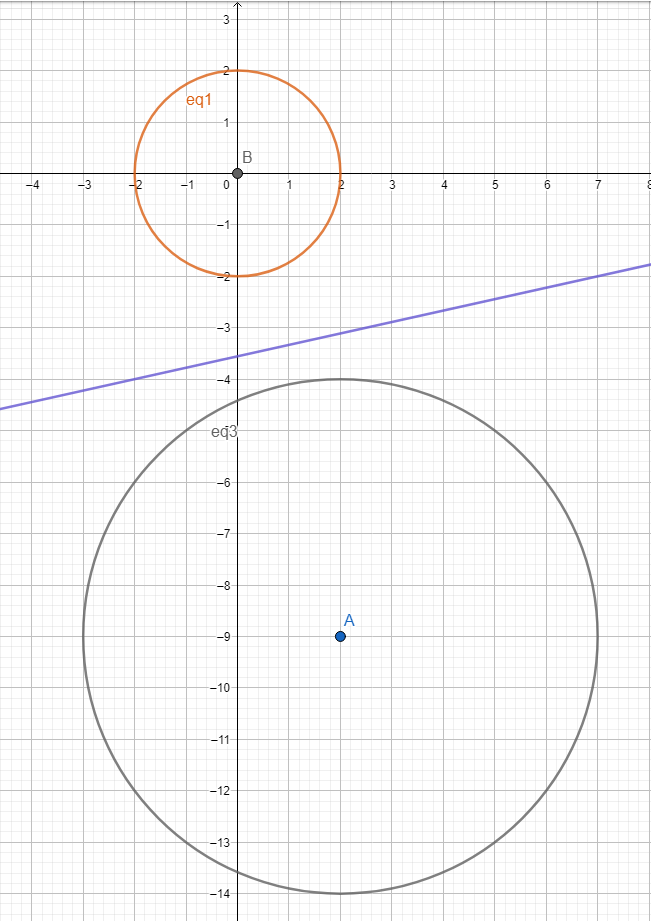## Cheat Sheet

• Be careful in dealing with the signs when the goal is to find the coordinates of the center when the equation of the circle is given.
• For instance, the circle ${\left(x+8\right)}^{2}+{\left(y-3\right)}^{2}=15$ has a center at $C\left(-8,3\right)$
• The circle ${\left(x-7\right)}^{2}+{\left(y-1\right)}^{2}=49$ has a center at $C\left(7,1\right)$.
• In finding the radius of the circle, always take the positive root. For instance, the circle ${\left(x+\frac{1}{2}\right)}^{2}+{\left(y-\frac{1}{4}\right)}^{2}=16$, then ${r}^{2}=16\to r=4$.
• The alternative formula to find the radius of the circle is ${r}^{2}=\frac{{B}^{2}+{C}^{2}-4AD}{4{A}^{2}}$.
• Use completing the square when transforming the general equation of a circle to its standard form ${\left(x-h\right)}^{2}+{\left(y-k\right)}^{2}={r}^{2}$

## Blunder Areas

• In a circle, $A=C$ in the equation $A{x}^{2}+C{y}^{2}+Dx+Ey+F=0$.
• In an ellipse, $AC>0$ in the equation $A{x}^{2}+C{y}^{2}+Dx+Ey+F=0$
• When the equation of a conic section is given, always know the difference between a circle and an ellipse.
• The discriminant of a circle is given by ${B}^{2}-4AC<0$ provided that .
• Do not be confused with the discriminant of an ellipse since it is also ${B}^{2}-AC<0$. The condition in this case is $AC>0$.
• In solving for the equation of the circle when the three points are given, we use  to generate systems of linear equations with D, E, and F as variables.